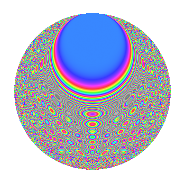# Properties

 Label 1575.2.buLevel 1575 Weight 2 Character orbit bu Rep. character $$\chi_{1575}(314,\cdot)$$ Character field $$\Q(\zeta_{10})$$ Dimension 320 Sturm bound 480

# Related objects

## Defining parameters

 Level: $$N$$ = $$1575 = 3^{2} \cdot 5^{2} \cdot 7$$ Weight: $$k$$ = $$2$$ Character orbit: $$[\chi]$$ = 1575.bu (of order $$10$$ and degree $$4$$) Character conductor: $$\operatorname{cond}(\chi)$$ = $$525$$ Character field: $$\Q(\zeta_{10})$$ Sturm bound: $$480$$

## Dimensions

The following table gives the dimensions of various subspaces of $$M_{2}(1575, [\chi])$$.

Total New Old
Modular forms 992 320 672
Cusp forms 928 320 608
Eisenstein series 64 0 64

## Trace form

 $$320q - 80q^{4} + O(q^{10})$$ $$320q - 80q^{4} - 80q^{16} + 40q^{22} - 16q^{25} - 60q^{28} + 56q^{46} - 16q^{49} + 120q^{58} - 32q^{64} - 52q^{70} + 16q^{79} + 8q^{85} + 68q^{91} + O(q^{100})$$

## Decomposition of $$S_{2}^{\mathrm{new}}(1575, [\chi])$$ into newform subspaces

The newforms in this space have not yet been added to the LMFDB.

## Decomposition of $$S_{2}^{\mathrm{old}}(1575, [\chi])$$ into lower level spaces

$$S_{2}^{\mathrm{old}}(1575, [\chi]) \cong$$ $$S_{2}^{\mathrm{new}}(525, [\chi])$$$$^{\oplus 2}$$

## Hecke Characteristic Polynomials

There are no characteristic polynomials of Hecke operators in the database